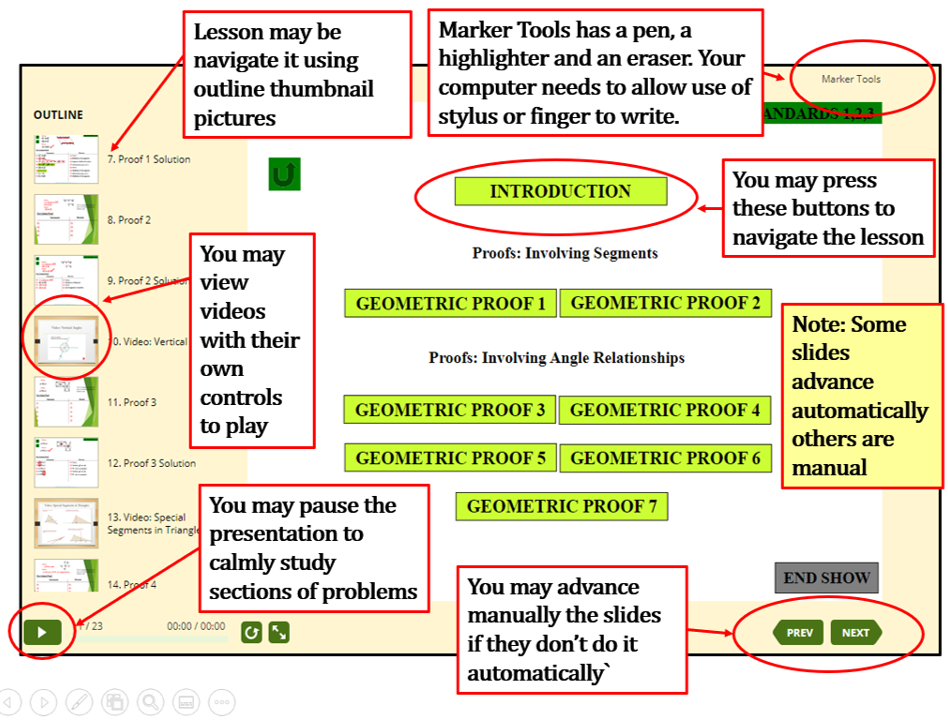QUICK SITE TOUR VIDEO OF HOW TO INTERACT

WITH THESE LESSONS (Click inside the graphic and Press Play Arrow Buttom)

# BASIC MATH TOPICS

Hands-on Approach from Concrete to Representional to Abstract

Scroll Down To Find the Lessons

 CHAPTER 1 EXPONENTS, POWERS, ROOTS AND FRACTIONS (ADD, SUBTRACT, MULTIPLY AND DIVIDE) 1.- Exponents: Powers, Roots, Scientific Notation and other topics. 2.- Add and Subract Fractions with Fraction Bars to Math Notation. 3.- Multiplying Fractions 4.- Proper and Improper Fractions a Conceptual Interpretation. 5.- Operations with Mixed Number a Conceptual Approach. 6.- Dividing Fractions. INSIDE EACH LESSON YOU MAY DO AS THIS GRAPHIC SHOWSCHAPTER 2 ADDING, SUBRACTING, MULTIPLYING AND DIVIDING DECIMALS 1.- Adding Decimals. 2.- Subracting Decimals. 3.- Multiplying Decimals. 4.- Dividing Decimals. CHAPTER 3 PERCENTS A CONCEPTUAL APPROACH APPLYING CONCEPTS IN CHAPTER 1 AND CHAPTER 2 1.- Convert a Fraction to a Percent. 2.- Percent of a Number. 3.- Comparing Numbers as Percent. 4.- Percent of Increase and Percent of Decrease. 5.- Amount of a Discount as Percent. 6.- Simple Interest.

HOME